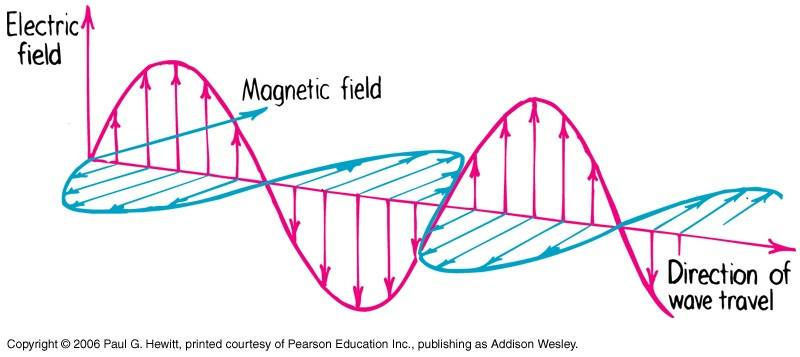# Relationship between E-field and Probability Amplitude of Waves

• I
In summary, the relationship between the electric field and the probability amplitude is that the intensity is proportional to the square of the E-field amplitude, and also proportional to the square of the probability amplitude. However, there is no direct proportionality between the two as one is a vector and the other is a scalar. The photon state is described by Fock states or its linear combination, while the electric field is associated with a field operator. The bold p in the Schrödinger equation for the photon represents momentum.

Electromagnetic waves can be classically described by Maxwell's equations.Photons can be described by probability waves.

In this case, what is the relationship between the electric field and the probability amplitude?

Are they directly proportional to each other? What about the fact that one is a vector and the other a scalar?

The Intensity is proportional to the square of the E-field amplitude, and also proportional to the square of the probability amplitude.

I thought this would be a commonly asked question.

Did you read the thread? Your question stated that photons could be described by probability waves and then asked a question that if that were were the case... The lack of responses would suggest that it cannot be the case.

Jilang said:
Did you read the thread? Your question stated that photons could be described by probability waves and then asked a question that if that were were the case... The lack of responses would suggest that it cannot be the case.

Yeah I know, but I was asking if you yourself know the answer.

A photon is not a non-relativistic object, this implies that its quantum behavior cannot be described by the (non-relativistic) Schroedinger equation. For non-relativistic particles, you can find its position representation wavefunction by solving the Schroedinger equation. Since you can't do that with photon, there is no spatial wavefunction in the usual sense of non-relativistic QM which can be associated to photons.